# Selina solutions for Concise Physics Class 10 ICSE chapter 8 - Current Electricity [Latest edition]

#### Chapters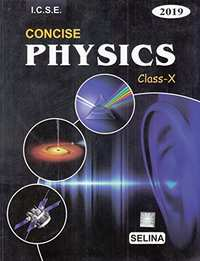## Solutions for Chapter 8: Current Electricity

Below listed, you can find solutions for Chapter 8 of CISCE Selina for Concise Physics Class 10 ICSE.

Exercise 8 (A) 1Exercise 8 (A) 2Exercise 8 (A) 3Exercise 8 (B) 1Exercise 8 (B) 2Exercise 8 (B) 3Exercise 8 (C) 1Exercise 8 (C) 2Exercise 8 (C) 3
Exercise 8 (A) 1 [Pages 186 - 187]

### Selina solutions for Concise Physics Class 10 ICSE Chapter 8 Current Electricity Exercise 8 (A) 1 [Pages 186 - 187]

Exercise 8 (A) 1 | Q 1 | Page 186

Define the term current and state its S.I unit.

Exercise 8 (A) 1 | Q 2 | Page 186

Define the term electric potential. State its S.I. unit.

Exercise 8 (A) 1 | Q 3 | Page 186

How is the electric potential difference between the two points defined? State its S.I. unit.

Exercise 8 (A) 1 | Q 4 | Page 186

Explain the statement ‘the potential difference between two points is 1 volt’.

Exercise 8 (A) 1 | Q 5.1 | Page 186

State whether the current is a scalar or vector? What does the direction of current convey?

Exercise 8 (A) 1 | Q 5.2 | Page 186

State whether the potential is a scalar or vector? What does the positive and negative sign of potential convey?

Exercise 8 (A) 1 | Q 7.1 | Page 186

Name the particles which are responsible for the flow of current in a metallic wire.

Exercise 8 (A) 1 | Q 7.2 | Page 186

Explain the flow of current in a metallic wire on the basis of movement of the particles named by you above in part.

Exercise 8 (A) 1 | Q 7.3 | Page 186

What is the cause of resistance offered by the metallic wire in the flow of current through it?

Exercise 8 (A) 1 | Q 8 | Page 186

State Ohm’s law and draw a neat labelled circuit diagram containing a battery, a key, a voltmeter, an ammeter, a rheostat and an unknown resistance to verify it.

Exercise 8 (A) 1 | Q 9 | Page 186

(a) Name and state the law which relates the potential difference and current in a conductor.

(b) What is the necessary condition for a conductor to obey the law named above in part (a) ?

Exercise 8 (A) 1 | Q 10 | Page 186

(a) draw a V-I graph for a conductor obeying Ohm’s law.

(b) what does the slope of V–I graph  for a conductor represent?

Exercise 8 (A) 1 | Q 11 | Page 186

Draw a I – V graph for a linear resistor. What does its slope represent?

Exercise 8 (A) 1 | Q 12 | Page 186

What is an ohmic resistor? Give one example of an ohmic resistor. Draw a graph to show its current voltage relationship. How is the resistance of the resistor determined from this graph?

Exercise 8 (A) 1 | Q 13 | Page 186

What are non-ohmic reistors? Give one example and draw a graph to show its current-voltage relationship.

Exercise 8 (A) 1 | Q 14 | Page 186

Give two difference between an ohmic and non-ohmic resistor.

Exercise 8 (A) 1 | Q 15 | Page 186

Figure , below shows the I-V characteristic curves for four resistors. Identify the ohmic and non-ohmic resistors. Give a reason for your answer.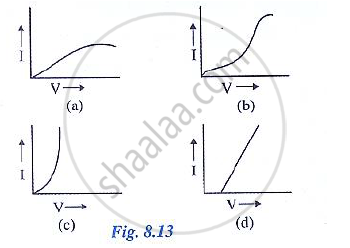Exercise 8 (A) 1 | Q 16 | Page 186

Draw a V-I graph for a conductor at two different temperature. What conclusion do you draw from your graph for the variation of resistance of conductor with temperature?

Exercise 8 (A) 1 | Q 17.1 | Page 186

Exercise 8 (A) 1 | Q 17.2 | Page 186

Two copper wires are of the same length, but one is thicker than the other.

1. Which wire will have more resistance?
2. Which wire will have more specific resistance?
Exercise 8 (A) 1 | Q 18 | Page 186

How does the resistance of a wire depend on its length? Give a reason for your answer with reason.

Exercise 8 (A) 1 | Q 19 | Page 186

How does the resistance of a metallic wire depend on its temperature? Explain with reason.

Exercise 8 (A) 1 | Q 20 | Page 186

Two wires one of copper and other of iron, are of the same length and same radius. Which will have more resistance? Give reason.

Exercise 8 (A) 1 | Q 21 | Page 186

Name three factors on which resistance of a given wire depends and state how is it affected by the factors stated by you.

Exercise 8 (A) 1 | Q 22 | Page 186

Define the term resistivity and state its S.I unit.

Exercise 8 (A) 1 | Q 23 | Page 186

Write an expression connecting the resistance and resistivity. State the meaning of symbols used.

Exercise 8 (A) 1 | Q 24 | Page 186

State the order of resistivity of (i) a metal, (ii) a semiconductor and (iii) an insulator.

Exercise 8 (A) 1 | Q 25.1 | Page 186

Name two factors on which the specific resistance of a wire depends?

Exercise 8 (A) 1 | Q 25.2 | Page 186

Two wires A and B are made of copper. The wire A is long and thin while the wire B is Short and thick. Which will have more specific resistance ?

Exercise 8 (A) 1 | Q 26 | Page 187

Name a substance of which the resistance remains almost unchanged by the increase in  temperature.

Exercise 8 (A) 1 | Q 27 | Page 187

How does specific resistance of a semi-conductor change with the increase in temperature?

Exercise 8 (A) 1 | Q 28 | Page 187

How does (a) resistance, and  (b) specific resistance of a wire depend on its (i) length, and (ii) radius?

Exercise 8 (A) 1 | Q 29 | Page 187

Name the material used for making the connection wires. Give a reason for your answer. Why
should a connection wire be thick?

Exercise 8 (A) 1 | Q 30 | Page 187

Name a material which is used for making the standard resistor. Give a reason for your answer.

Exercise 8 (A) 1 | Q 31 | Page 187

Name the material used for making a fuse wire. Give a reason.

Exercise 8 (A) 1 | Q 32.1 | Page 187

Name the material used for  filament of an electric bulb .

Exercise 8 (A) 1 | Q 32.2 | Page 187

Name the material used for heating element of a room heater.

Exercise 8 (A) 1 | Q 33 | Page 187

What is a superconductor? Give one example of it.

Exercise 8 (A) 1 | Q 34 | Page 187

A substance has zero resistance below 1 k. what is such a substance called?

Exercise 8 (A) 2 [Page 187]

### Selina solutions for Concise Physics Class 10 ICSE Chapter 8 Current Electricity Exercise 8 (A) 2 [Page 187]

Exercise 8 (A) 2 | Q 1 | Page 187

Which of the following is an ohmic resistance?

• LED

• Junction diode

• Filament of a bulb

• Nichrome wire

Exercise 8 (A) 2 | Q 2 | Page 187

For which of the following substance, resistance decreases with increase in temperature?

• Copper

• Mercury

• Carbon

• Platinum

Exercise 8 (A) 3 [Page 187]

### Selina solutions for Concise Physics Class 10 ICSE Chapter 8 Current Electricity Exercise 8 (A) 3 [Page 187]

Exercise 8 (A) 3 | Q 1 | Page 187

In a conductor 6.25 × 10^16 electrons flow from its end A to B in 2 s. Find the current flowing
through the conductor (e = 1.6 × 10^19 C)

Exercise 8 (A) 3 | Q 2 | Page 187

A current of 1.6 mA flows through a conductor. If charge on an electron is – 1.6 × 10^-19 coulomb,
find the number of electrons that will pass each second through the cross section of that
conductor.

Exercise 8 (A) 3 | Q 3 | Page 187

Find the potential difference required to pass a current of 0.2 A in a wire of resistance 20Ω

Exercise 8 (A) 3 | Q 4 | Page 187

An electric bulb draws 1.2 A current at 6.0 V. Find the resistance of filament of bulb while glowing.

Exercise 8 (A) 3 | Q 5 | Page 187

A car bulb connected to a 12 volt battery draws 2 A current when glowing. What is the resistance of the filament of the bulb? Will the resistance be more same or less when the bulb is not glowing?

Exercise 8 (A) 3 | Q 6 | Page 187

Calculate the current flowing through a wire of resistance 5 Ω connected to a battery of potential difference 3 V.

Exercise 8 (A) 3 | Q 7 | Page 187

In an experiment of verification of Ohm’s law following observations are obtained.

 potential difference V (in volt) 0.5 1 1.5 2 2.5 current I (in ampere) 0.2 0.4 0.6 0.8 1

Draw a characteristic V-I graph and use this graph to find:

1. potential difference V when the current I is 0.5 A,
2. current I when the potential difference V is 0.75 V,
3. resistance in a circuit.
Exercise 8 (A) 3 | Q 8 | Page 187

Two wires of the same material and same length have radii r1 and r2 respectively compare: (i)
their resistances, (ii) their resistivities.

Exercise 8 (A) 3 | Q 9 | Page 187

A given wire of resistance 1 Ω is stretched to double its length. What will be its new resistance?

Exercise 8 (A) 3 | Q 10 | Page 187

A wire 3 ohm resistance and 10 cm length is stretched to 30 cm length. Assuming that it has a
uniform cross section, what will be its new resistance?

Exercise 8 (A) 3 | Q 11 | Page 187

A wire of 9 ohm resistance having 30 cm length is tripled on itself. What is its new resistance?

Exercise 8 (A) 3 | Q 12 | Page 187

What length of copper wire of resistivity 1.7 × 10-8 𝛀 m and radius 1 mm is required so that its resistance is 1𝛀?

Exercise 8 (A) 3 | Q 13 | Page 187

The filament of a bulb takes a current 100 mA when potential difference across it is 0.2 V. When the potential difference across it becomes 1.0 V, the current becomes 400 mA. Calculate the resistance of filament in each case and account for the difference.

Exercise 8 (B) 1 [Page 200]

### Selina solutions for Concise Physics Class 10 ICSE Chapter 8 Current Electricity Exercise 8 (B) 1 [Page 200]

Exercise 8 (B) 1 | Q 1 | Page 200

Explain the meaning of the terms e.m.f.., terminal voltage, and internal resistance of a cell.

Exercise 8 (B) 1 | Q 2 | Page 200

State two differences between the e.m.f and terminal voltage of a cell.

Exercise 8 (B) 1 | Q 3 | Page 200

Name two factors on which the internal resistance of a cell depends and state how does it depend on the factors stated by you.

Exercise 8 (B) 1 | Q 4 | Page 200

A cell of e.m.f ε and internal resistance r is used to send current to an external resistance R. write expresssions for (a) the total resistance of circuit, (b) the current drawn from the cell. (c) the p.d
across the cell. And (d) voltage drop inside the cell.

Exercise 8 (B) 1 | Q 5 | Page 200

A cell is used to send current to an external circuit. (a) How does the voltage across its terminals compare with its e.m.f? (b) under what condition is the e.m.f of a cell equal to its terminal
voltage?

Exercise 8 (B) 1 | Q 6 | Page 200

Explain why the p.d across the terminals of a cell is more in an open circuit and reduced in a closed circuit.

Exercise 8 (B) 1 | Q 7 | Page 200

Write the expressions for the equivalent resistance R of three resistors R1, R2 and R3 joined in
(a) parallel (b) series

Exercise 8 (B) 1 | Q 8 | Page 200

How would you connect two resistors in series? Draw a diagram. Calculate the total equivalent resistance.

Exercise 8 (B) 1 | Q 9 | Page 200

Show by a diagram how two resistors R1 and R2 are joined in parallel. Obtain an expression for the total resistance of combination.

Exercise 8 (B) 1 | Q 10.1 | Page 200

State how are the two resistors joined with a battery in each of the following cases when:

same current flows in each resistor

Exercise 8 (B) 1 | Q 10.2 | Page 200

State how are the two resistors joined with a battery in each of the following cases when:

potential difference is same across each resistor

Exercise 8 (B) 1 | Q 10.3 | Page 200

State how are the two resistors joined with a battery in each of the following cases when:

equivalent resistance is less than either of the two resistances

Exercise 8 (B) 1 | Q 10.4 | Page 200

State how are the two resistors joined with a battery in each of the following cases when:

equivalent resistance is more than either of the two resistances.

Exercise 8 (B) 1 | Q 11 | Page 200

The V-I graph for a series combination and for a parallel combination of two resistors is shown in Fig – 8.38. Which of the two, A or B, represents the parallel combination? Give a reason for your answer.

Exercise 8 (B) 2 [Page 200]

### Selina solutions for Concise Physics Class 10 ICSE Chapter 8 Current Electricity Exercise 8 (B) 2 [Page 200]

Exercise 8 (B) 2 | Q 1 | Page 200

In series combination of resistances:

• P.d. is same across each resistance

• Total resistance is reduced

• Current is same in each resistance

• All of the above are true

Exercise 8 (B) 2 | Q 2 | Page 200

In parallel combination of resistances:

• P.D. is same across each resistance

• Total resistance is increased

• Current is same in each resistance

• All of the above are true

Exercise 8 (B) 2 | Q 3 | Page 200

Which of the following combinations have the same equivalent resistance between X and Y?

•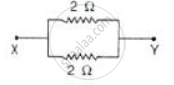•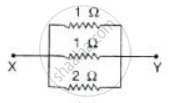•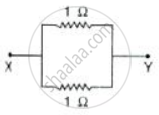•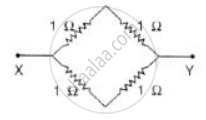Exercise 8 (B) 3 [Pages 201 - 203]

### Selina solutions for Concise Physics Class 10 ICSE Chapter 8 Current Electricity Exercise 8 (B) 3 [Pages 201 - 203]

Exercise 8 (B) 3 | Q 1 | Page 201

The diagram below in Fig. 8.40 shows a cell of e.m.f. ε = 2 volt and internal resistance r = 1 ohm to an external resistance R = 4 ohm. The ammeter A measures the current in the circuit and the
voltmeter V measures the terminal voltage across the cell. What will be the readings of the ammeter and voltmeter when (i) the key K is open, (ii) the key K is closed.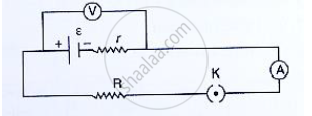Exercise 8 (B) 3 | Q 2 | Page 201

A battery of e.m.f 3.0 V supplies current through a circuit in which the resistance can be changed.
A high resistance voltmeter is connected across the battery. When the current is 1.5 A, the voltmeter reads 2.7 V. Find the internal resistance of the battery.

Exercise 8 (B) 3 | Q 3 | Page 201

A cell of e.m.f. 1.8V and internal resistance 2Ω is connected in series with an ammeter of resistance 0.7Ω and a resistor of 4.5Ω as shown in Fig. 8.41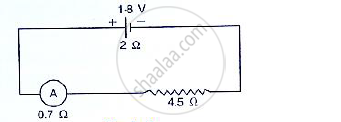(a) what would be the reading of the ammeter?
(b) what is the potential difference across the terminals of the cell?

Exercise 8 (B) 3 | Q 4 | Page 201

A battery of e.m.f. 15 V and internal resistance 3 ohm is connected to two resistors of resistances 3 ohm and 6 ohm is series Find:
(a) the current through the battery
(b) the p.d. between the terminals of the battery.

Exercise 8 (B) 3 | Q 5 | Page 201

A cell of e.m.f. ε and internal resistance 𝔯 sends current 1.0 A when it is connected to an external resistance 1.9Ω. But it sends current 0.5 A when it is connected to an external resistance 3.9 Ω.
Calculate the values of ε and 𝔯.

Exercise 8 (B) 3 | Q 6 | Page 201

Two resistors having resistance 4𝛀 and 6𝛀 are connected in parallel. Find their equivalent resistance.

Exercise 8 (B) 3 | Q 7 | Page 201

Four resistors each of resistance 2Ω are connected in parallel. What is the effective resistance?

Exercise 8 (B) 3 | Q 8 | Page 201

You have three resistors of values 2Ω, 3Ω and 5Ω. How will you join them so that the total  resistance is less than 1Ω? Draw diagram and find the total resistance.

Exercise 8 (B) 3 | Q 9 | Page 201

Three resistors each of 2Ω are connected together so that their total resistance is 3Ω. Draw a diagram to show this arrangement and check it by calculation.

Exercise 8 (B) 3 | Q 10 | Page 201

Calculate the equivalent resistance of the following combination of resistors r1, r2, r3 and r4 if r_1=r_2=r_3=r_4=2.0Ω  between the points A and B in Fig. 8.42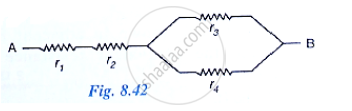Exercise 8 (B) 3 | Q 11 | Page 201

A combination consists of three resistors in series. Four similar sets are connected in parallel. If the resistance of each resistor is 2 ohm, find the resistance of the combination.

Exercise 8 (B) 3 | Q 12 | Page 201

In the circuit shown below in Fid 8.43, calculate the value of x if the equivalent resistance between A and B is 4Ω.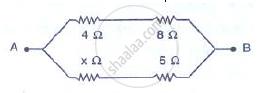Exercise 8 (B) 3 | Q 13 | Page 201

Calculate the effective resistance between the points A and B in the circuit shown in Figure 8.44.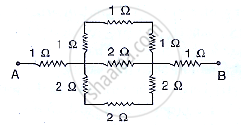Exercise 8 (B) 3 | Q 14 | Page 202

A wire of uniform thickness with a resistance of 27Ω is cut into three equal pieces and they are joined in parallel. Find the equivalent resistance of the parallel combination.

Exercise 8 (B) 3 | Q 15 | Page 202

A circuit consists of a 1 ohm resistor in series with a parallel arrangement of 6 ohm and 3 ohm resistors. Calculate the total resistance if the circuit. Draw a diagram.

Exercise 8 (B) 3 | Q 16 | Page 202

Calculate the effective resistance between the points A and B in the network shown below in figure.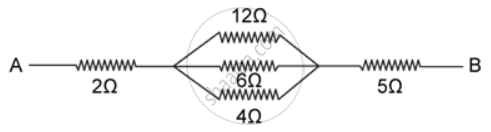Exercise 8 (B) 3 | Q 17 | Page 202

Calculate the equivalent resistance between A and B in the adjacent diagram.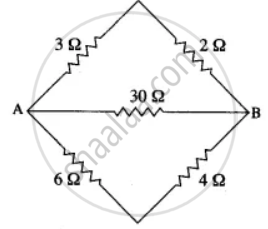Exercise 8 (B) 3 | Q 18 | Page 202

In the network shown in the following adjacent Figure, calculate the equivalent resistance between the points.

1. A and B
2. A and C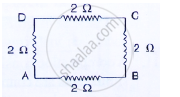Exercise 8 (B) 3 | Q 19 | Page 202

Five resistors, each 3 Ω, are connected as shown in Fig 8.48. Calculate the resistance (a) between the points P and Q. (b) between the points X and Y.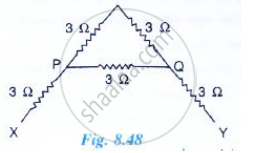Exercise 8 (B) 3 | Q 20 | Page 202

Two resistors of 2.0 Ω and 3.0 Ω are connected (a) in series (b) in parallel, with a battery of 6.0 V and negligible internal resistance. For each case draw a circuit diagram and calculate the current through the battery.

Exercise 8 (B) 3 | Q 21 | Page 202

A resistor of 6Ω is connected in series with another resistor of 4 Ω. A potential difference of 20 V is applied across the combination. Calculate (a) the current in the circuit and (b) the potential difference across the 6Ω resistor.

Exercise 8 (B) 3 | Q 22.1 | Page 202

Two resistors of resistance 4 Ω and 6 Ω are connected in parallel to a cell to draw 0.5 A current from the cell.

Draw a labeled diagram of the arrangement

Exercise 8 (B) 3 | Q 22.2 | Page 202

Two resistors of resistance 4 Ω and 6 Ω are connected in parallel to a cell to draw 0.5 A current from the cell.

Calculate current in each resistor.

Exercise 8 (B) 3 | Q 23 | Page 202

Calculate current flowing through each of the resistors A and B in the circuit shown in following figure?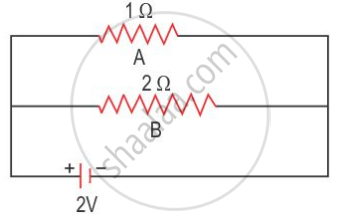Exercise 8 (B) 3 | Q 24 | Page 202

In the following figure calculate:

1. the total resistance of the circuit
2. the value if R, and
3. the current flowing in R.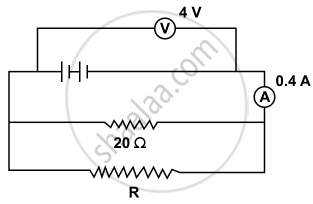Exercise 8 (B) 3 | Q 25.1 | Page 202

A particular resistance wire has a resistance of 3 ohm per meter. Find:
The total resistance of three lengths of this wire each 1.5 m long, joined in parallel.

Exercise 8 (B) 3 | Q 25.2 | Page 202

A particular resistance wire has a resistance of 3 ohm per meter. Find :

The potential difference of the battery which gives a current of 2 A in each of the 1.5 m length when connected in the parallel to the battery (assume that resistance of the battery is negligible).

Exercise 8 (B) 3 | Q 25.3 | Page 203

A particular resistance wire has a resistance of 3 ohm per meter. Find :

The total resistance of three lengths of this wire each 1.5 m long, in parallel.

Exercise 8 (B) 3 | Q 26 | Page 203

A cell supplies a current of 1.2 A through two 2 Ω resistors connected in parallel. When the resistors are connected in series, it supplies a current of 0.4 A. Calculate: (i) the internal resistance and (ii) e.m.f. of the cell.

Exercise 8 (B) 3 | Q 27 | Page 203

A battery of e.m.f 15 V and internal resistance 3 Ω is connected to two resistors 3Ω and 6Ω connected in parallel. Find: (a) the current through the battery. (b) p.d. between the terminals of the battery, (c) the current in 3 Ω resistors, (d) the current in 6 Ω resistor.

Exercise 8 (B) 3 | Q 28 | Page 203

The following circuit diagram shows three resistors 2 Ω, 4 Ω and R Ω connected to a battery of e.m.f 2V and internal resistance 3 Ω. If the main current of 0.25 A flows through the circuit, find:

1. the p.d. across the 4 Ω resistors,
2. the p.d. across the internal resistance of the cell,
3. the p.d. across the R Ω or 2 Ω resistors, and
4. the value of R.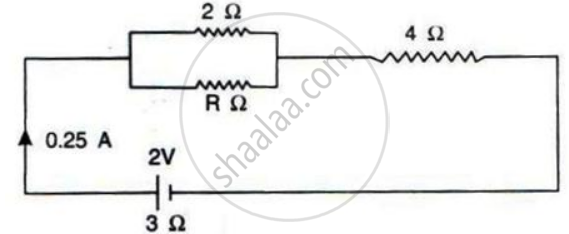Exercise 8 (B) 3 | Q 29 | Page 203

Three resistors of 6.0 ohm, 2.0 ohm and 4.0 ohm are joined to ammeter A and a cell of emf 6.0 V as shown in following figure. Calculate :

(a) the efective resistance of the circuit.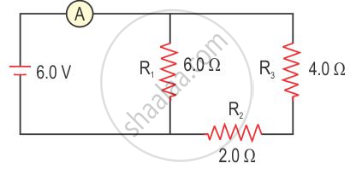Exercise 8 (B) 3 | Q 30 | Page 203

The following diagram shows the arrangement of five different resistances connected to a battery of e.m.f. 1.8V Calculate:
(a) the total resistance of the circuit, and
(b) the reading of ammeter A.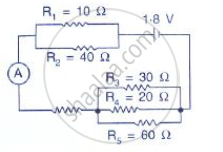Exercise 8 (B) 3 | Q 31 | Page 203

A cell of e.m.f. 2 V and internal resistance 1.2 Ω is connected to an ammeter of resistance 0.8 Ω and two resistors of 4.5 Ω and 9 Ω as shown in following figure.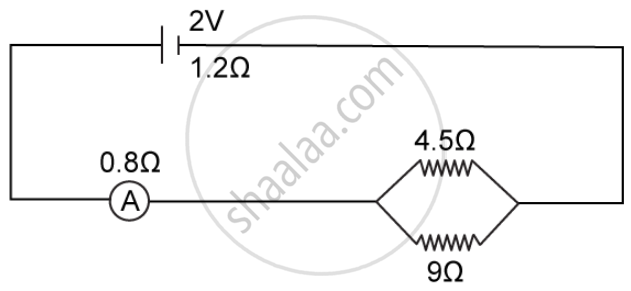Find:

1. The reading of the ammeter,
2. The potential difference across the terminals of the cells, and
3. The potential difference across the 4.5 Ω resistor.
Exercise 8 (C) 1 [Pages 211 - 212]

### Selina solutions for Concise Physics Class 10 ICSE Chapter 8 Current Electricity Exercise 8 (C) 1 [Pages 211 - 212]

Exercise 8 (C) 1 | Q 1 | Page 211

Write an expression for the electrical energy spent in flow of current through an electrical appliance in terms of current, resistance and time.

Exercise 8 (C) 1 | Q 2.1 | Page 211

Write an expression for the electrical power spent in flow of current through a conductor in terms of resistance and potential difference.

Exercise 8 (C) 1 | Q 2.2 | Page 211

Write an expression for the electrical power spent in flow of current through a conductor in terms of  current and resistance.

Exercise 8 (C) 1 | Q 3 | Page 211

Electrical power P is given by the expression P = (Q × V) ÷ time

(a) What do the symbols Q and V represent?

(b) Express the power P in terms of current and resistance explaining the meanings of symbols used there in.

Exercise 8 (C) 1 | Q 4 | Page 211

Name the S.I. unit of electrical energy. How is it related to Wh?

Exercise 8 (C) 1 | Q 5 | Page 211

Explain the meaning of the statement 'the power of an appliance is 100 W'.

Exercise 8 (C) 1 | Q 6 | Page 211

State the S.I. units of electrical power.

Exercise 8 (C) 1 | Q 7.1 | Page 212

State and define the household unit of electricity.

Exercise 8 (C) 1 | Q 7.2 | Page 212

What is the voltage of the electricity that is generally supplied to a house?

Exercise 8 (C) 1 | Q 7.3 | Page 212

What is consumed while using different electrical appliances, for which electricity bills are paid?

Exercise 8 (C) 1 | Q 8 | Page 212

Name the physical quantity which is measured in (i) kW, (ii) kWh.

Exercise 8 (C) 1 | Q 9 | Page 212

Define the term kilowatt - hour and state its value in S.I. unit.

Exercise 8 (C) 1 | Q 10 | Page 212

How do kilowatt and kilowatt-hour differ ?

Exercise 8 (C) 1 | Q 11.1 | Page 212

Complete the following:

1 kWh = (1 "volt" xx 1 "ampere" xx "_________")/1000

Exercise 8 (C) 1 | Q 11.2 | Page 212

Complete the following:

1 kWh = _________ J

Exercise 8 (C) 1 | Q 12 | Page 212

What do you mean by power rating of an electrical appliance? How do you use it to calculate

(a) the resistance of the appliance and

(b) the safe limit of the current in it, while in use?

Exercise 8 (C) 1 | Q 13 | Page 212

An electric bulb is marked '100 W, 250 V'. What information does this convey?

Exercise 8 (C) 1 | Q 14 | Page 212

List the names of three electrical gadgets used in your house. Write their power, voltage rating and approximate time for which each one is used in a day. Hence find the electrical energy consumed by each in day.

Exercise 8 (C) 1 | Q 15 | Page 212

Twolamps, one rated 220 V, 50 W and the other rated 220 V, 100 W, are connected in series with mains of 220 V. Explain why does the 50 W lamp consume more power.

Exercise 8 (C) 1 | Q 16 | Page 212

Name the factors on which the heat produced in a wire depends when current is passed in it, and state how does it depend on the factors stated by you.

Exercise 8 (C) 2 [Page 212]

### Selina solutions for Concise Physics Class 10 ICSE Chapter 8 Current Electricity Exercise 8 (C) 2 [Page 212]

Exercise 8 (C) 2 | Q 1 | Page 212

When a current I flows through a resistance R for time t, the electrical energy spent is given by :

• IRt

• I2Rt

• IR2t

• I2R/t

Exercise 8 (C) 2 | Q 2 | Page 212

An electrical appliance has a rating 100 W, 120V. The resistance of element of appliance when in use:

• 1.2 Ω

• 144 Ω

• 120 Ω

• 100 Ω

Exercise 8 (C) 3 [Pages 212 - 213]

### Selina solutions for Concise Physics Class 10 ICSE Chapter 8 Current Electricity Exercise 8 (C) 3 [Pages 212 - 213]

Exercise 8 (C) 3 | Q 1 | Page 212

An electric bulb of resistance 500 Ω draws current 0.4 A from the source. Calculate:
(a) the power of bulb and
(b) the potential difference at its end.

Exercise 8 (C) 3 | Q 2 | Page 212

A current of 2 A is passed through a coil of resistance 75 Ω for 2 minutes.
(a) How much heat energy is produced?
(b) How much charge is passed through the resistance?

Exercise 8 (C) 3 | Q 3 | Page 212

Calculate the current through a 60 W lamp rated 250 V. If the line voltage falls to 200 V, how is power consumed by the bulb affected?

Exercise 8 (C) 3 | Q 4 | Page 212

An electric bulb is rated '100 W, 250 V'. How much current will the bulb draw if connected to a 250 V supply ?

Exercise 8 (C) 3 | Q 5 | Page 212

An electric bulb is rated at 220 V, 100 W.
(a) What is its resistance?
(b) What safe current can be passed through it?

Exercise 8 (C) 3 | Q 6 | Page 212

A bulb of 40 W is used for 12.5 h each day for 30 days. Calculate the electrical energy consumed.

Exercise 8 (C) 3 | Q 7 | Page 212

An electric iron is rated 750 W, 230 V. Calculate the electrical energy consumed by the iron in 16 hours.

Exercise 8 (C) 3 | Q 8.1 | Page 212

An electrical appliance having a resistance of 200 Ω  is operated at 220 V. calculate the energy consumed by the appliance in 5 minutes.
in joules.

Exercise 8 (C) 3 | Q 8.2 | Page 212

An electrical appliance having a resistance of 200 Ω is operated at 220 V. calculate the energy consumed by the appliance in 5 minutes in kWh.

Exercise 8 (C) 3 | Q 9 | Page 212

A bulb marked 12 V, 24 W operates on a 12 V battery for 20 minutes. Calculate:

(i) The current flowing through it, and

(ii) The energy consumed.

Exercise 8 (C) 3 | Q 10 | Page 212

A current of 0.2 A flows through a wire whose ends are at a potential difference of 15 V. Calculate:

(i) The resistance of the wire, and

(ii) The heat energy produced in 1 minute.

Exercise 8 (C) 3 | Q 11 | Page 212

What is the resistance, under normal working conditions, of an electric lamp rated at '240 v, 60 W' ? If two such lamps are connected in series across a 240 V mains supply, explain why each one appears less bright.

Exercise 8 (C) 3 | Q 12 | Page 213

Two bulbs are rated 60 W, 220 V and 60 W, 110 V respectively. Calculate the ratio of their resistances.

Exercise 8 (C) 3 | Q 13 | Page 213

An electric bulb is rated 250 W, 230 V.

(i) the energy consumed in one hour, and

(ii) the time in which the bulb will consume 1.0 kWh energy when connected to 230 V mains.

Exercise 8 (C) 3 | Q 14.1 | Page 213

Three heaters each rated 250 W, 100 V are connected in parallel to a 100 V supply. Calculate :

The total current taken from the supply

Exercise 8 (C) 3 | Q 14.2 | Page 213

Three heaters each rated 250 W, 100 V are connected in parallel to a 100 V supply. The resistance of each heater

Exercise 8 (C) 3 | Q 14.3 | Page 213

Three heaters each rated 250 W, 100 V are connected in parallel to a 100 V supply of the energy supplied in kWh to the three heaters in 5 hours.

Exercise 8 (C) 3 | Q 15.1 | Page 213

A bulb is connected to a battery of p.d. 4 V and internal resistance 2.5 Ω . A steady current of 0.5 A flows through the circuit. Calculate:

The total energy supplied by the battery in 10 minutes

Exercise 8 (C) 3 | Q 15.2 | Page 213

A bulb is connected to a battery of p.d. 4 V and internal resistance 2.5 Ω . A steady current of 0.5 A flows through the circuit. Calculate:

The resistance of the bulb

Exercise 8 (C) 3 | Q 15.3 | Page 213

A bulb is connected to a battery of p.d. 4 V and internal resistance 2.5 Ω . A steady current of 0.5 A flows through the circuit. Calculate:

The energy dissipated in the bulb in 10 minutes.

Exercise 8 (C) 3 | Q 16.1 | Page 213

Two resistors A and B of 4 Ω and 6 Ω  respectively are connected in parallel. The combination is connected across a 6 volt battery of negligible resistance. Calculate: the power supplied by the battery .

Exercise 8 (C) 3 | Q 16.2 | Page 213

Two resistors A and B of 4 Ω and 6 Ω respectively are connected in parallel. The combination is connected across a 6 volt battery of negligible resistance. Calculate :

the power dissipated in each resistor.

Exercise 8 (C) 3 | Q 17 | Page 213

A battery of e.m.f. 15 V and internal resistance 2 Ω   is connected to two resistors of resistances 4 ohm and 6 ohm joined (a) in series. Find in each case the electrical energy spent per minute in 6 Ω resistor.

Exercise 8 (C) 3 | Q 18 | Page 213

Water in an electric kettle connected to a 220 V supply took 5 minutes to reach its boiling point. How long would it have taken if the supply had been of 200 V?

Exercise 8 (C) 3 | Q 19 | Page 213

An electric toaster draws 8 A current in a 220 V circuit. It is used for 2 hr. Find the cost of operating the toaster if the cost of electrical energy is Rs. 4.50 per kWh.

Exercise 8 (C) 3 | Q 20 | Page 213

An electric kettle is rated 2.5 kW, 250 V. Find the cost of running the kettle for two hours at Rs. 5.40 per unit.

Exercise 8 (C) 3 | Q 21 | Page 213

A geyser is rated 1500 W, 250 V. This geyser is connected to 250 V mains. Calculate:

(i) The current drawn,

(ii) The energy consumed in 50 hours, and

(iii) The cost of energy consumed at Rs 4.20 per kWh.

## Solutions for Chapter 8: Current Electricity

Exercise 8 (A) 1Exercise 8 (A) 2Exercise 8 (A) 3Exercise 8 (B) 1Exercise 8 (B) 2Exercise 8 (B) 3Exercise 8 (C) 1Exercise 8 (C) 2Exercise 8 (C) 3## Selina solutions for Concise Physics Class 10 ICSE chapter 8 - Current Electricity

Shaalaa.com has the CISCE Mathematics Concise Physics Class 10 ICSE CISCE solutions in a manner that help students grasp basic concepts better and faster. The detailed, step-by-step solutions will help you understand the concepts better and clarify any confusion. Selina solutions for Mathematics Concise Physics Class 10 ICSE CISCE 8 (Current Electricity) include all questions with answers and detailed explanations. This will clear students' doubts about questions and improve their application skills while preparing for board exams.

Further, we at Shaalaa.com provide such solutions so students can prepare for written exams. Selina textbook solutions can be a core help for self-study and provide excellent self-help guidance for students.

Concepts covered in Concise Physics Class 10 ICSE chapter 8 Current Electricity are Resistance (R), Ohmic and Non-ohmic Resistors, Choice of Material of a Wire, Superconductors, Electro-motive Force (E.M.F.) of a Cell, Terminal Voltage of a Cell, Internal Resistance of a Cell, Experimental Verification of Ohm’s Law, Electric Current, System of Resistors, Resistances in Parallel, Electric Circuit, Electric Charge, Limitations of Ohm’s Law, Ohm's Law (V = IR), Resistors in Series, Combination of Resistors Both in Series and Parallel, Potential and Potential Difference, Electrical Power, Electrical Energy, Electrical Resistivity and Electrical Conductivity, Measurement of Electrical Energy (Expression W = QV = Vlt), Commercial Unit of Electrical Energy, Power Rating of Appliances, Household Consumption of Electric Energy, Effects of Electric Current, Heating Effect of Electric Current, Factors Affecting the Resistance of a Conductor.

Using Selina Concise Physics Class 10 ICSE solutions Current Electricity exercise by students is an easy way to prepare for the exams, as they involve solutions arranged chapter-wise and also page-wise. The questions involved in Selina Solutions are essential questions that can be asked in the final exam. Maximum CISCE Concise Physics Class 10 ICSE students prefer Selina Textbook Solutions to score more in exams.

Get the free view of Chapter 8, Current Electricity Concise Physics Class 10 ICSE additional questions for Mathematics Concise Physics Class 10 ICSE CISCE, and you can use Shaalaa.com to keep it handy for your exam preparation.

Share